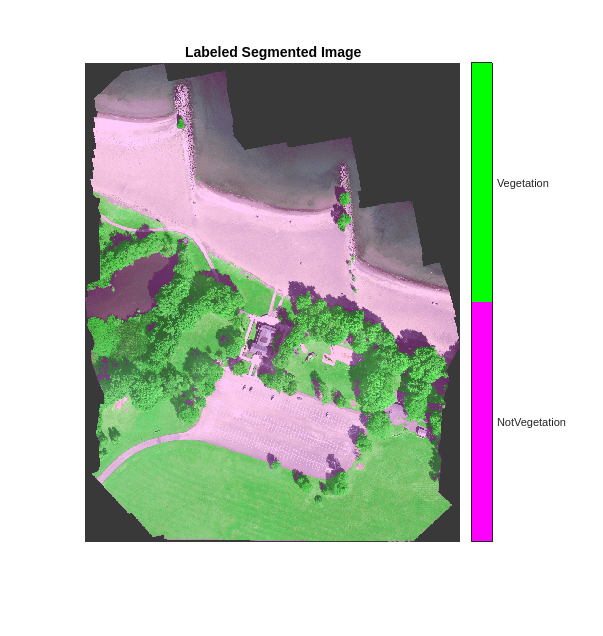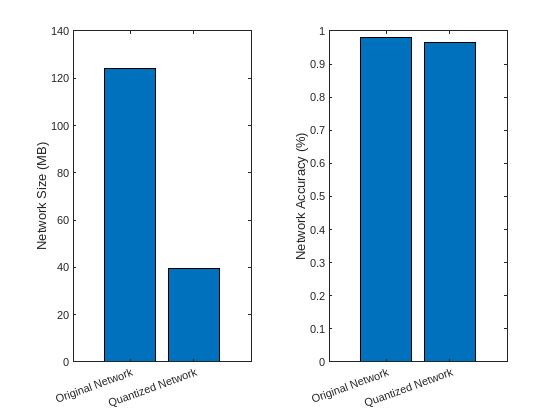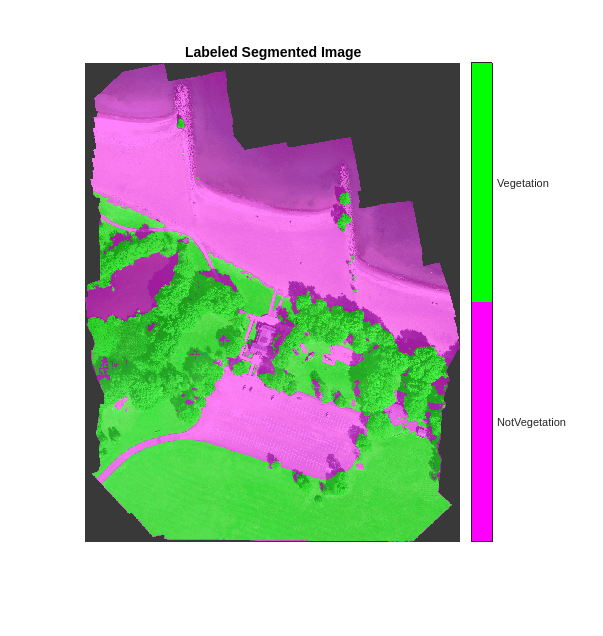# Quantize Semantic Segmentation Network and Generate CUDA Code

This example shows how to quantize a pretrained network for semantic segmentation and generate CUDA® code for deploying the network to a GPU environment.

Semantic segmentation involves labeling each pixel in an image with a class. One application of semantic segmentation is tracking deforestation, which is the change in forest cover over time. Environmental agencies track deforestation to assess and quantify the environmental and ecological health of a region.

Deep learning based semantic segmentation can yield a precise measurement of vegetation cover from high-resolution aerial photographs. One challenge is differentiating classes with similar visual characteristics, such as trying to classify a green pixel as grass, shrubbery, or tree. To increase classification accuracy, some data sets contain multispectral images that provide additional information about each pixel. For example, the Hamlin Beach State Park data set supplements the color images with three near-infrared channels that provide a clearer separation of the classes.Neural networks use memory to store input data, parameters (weights), and activations from each layer as the input propagates through the network. Most neural networks that you create and train using Deep Learning Toolbox™ use single-precision floating-point data types. Even small networks require a considerable amount of memory and hardware to perform these floating-point arithmetic operations. These restrictions can inhibit deployment of deep learning models to devices that have low computational power and less memory resources. By using a lower precision to store the weights and activations, you can reduce the memory requirements of the network.

In this example, you use the Deep Learning Toolbox in tandem with the Deep Learning Toolbox Model Quantization Library support package to reduce the memory footprint of a deep neural network by quantizing the weights, biases, and activations of convolution layers to 8-bit scaled integer data types. You then quantize the network in MATLAB® and test its performance by simulating quantized inference behavior. Then, you can optionally use GPU Coder™ to generate CUDA code for the optimized network. The generated code takes advantage of NVIDIA® CUDA deep neural network library (cuDNN) or the TensorRT™ high performance inference library. The generated code can be integrated into your project as source code, static or dynamic libraries, or executables that you can deploy to a variety of NVIDIA GPU platforms.

The network in this example is a U-Net, trained to perform semantic segmentation of a multispectral image with seven channels. For more information about semantic segmentation using U-Net, see Semantic Segmentation of Multispectral Images Using Deep Learning (Image Processing Toolbox).

This example uses the RIT-18 data set which contains high-resolution multispectral image data captured using a drone over the Hamlin Beach State Park, NY . The data contains labeled training, validation, and test sets, with 18 object class labels. The size of the data set is 3.0 GB.

Download the data set as a MAT file using the `downloadHamlinBeachMSIData` function. This function is attached to the example as a supporting file. To access this file, open the example as a live script. Specify `destination` as the desired location of the data.

```destination = fullfile(pwd); url = "https://home.cis.rit.edu/~cnspci/other/data/rit18_data.mat"; downloadHamlinBeachMSIData(url,destination);```

The multispectral image data is arranged as numChannels-by-width-by-height arrays. However, in MATLAB®, multichannel images are arranged as width-by-height-by-numChannels arrays. To reshape the data so that the channels are in the third dimension, use the `switchChannelsToThirdPlane` function. This function is attached to the example as a supporting file. Load the data set and reshape the images.

```load(fullfile(destination,"rit18_data","rit18_data.mat")); train_data = switchChannelsToThirdPlane(train_data); val_data = switchChannelsToThirdPlane(val_data); test_data = switchChannelsToThirdPlane(test_data); whos train_data val_data test_data```
``` Name Size Bytes Class Attributes test_data 12446x7654x7 1333663576 uint16 train_data 9393x5642x7 741934284 uint16 val_data 8833x6918x7 855493716 uint16 ```

Create a vector of class names.

```classNames = [ "RoadMarkings","Tree","Building","Vehicle","Person", ... "LifeguardChair","PicnicTable","BlackWoodPanel",... "WhiteWoodPanel","OrangeLandingPad","Buoy","Rocks",... "LowLevelVegetation","Grass_Lawn","Sand_Beach",... "Water_Lake","Water_Pond","Asphalt"]; ```

Save the training image data and validation image data as MAT files and the training labels as a PNG file. This facilitates loading the data using `imageDatastore` and `pixelLabelDatastore` objects.

```save("train_data.mat","train_data"); save("val_data.mat","val_data") imwrite(train_labels,"train_labels.png");```

Download a pretrained U-Net network and inspect some of its layers. The network was trained using single-precision floating-point data.

```trainedUnet_url = "https://www.mathworks.com/supportfiles/vision/data/multispectralUnet.mat"; downloadTrainedUnet(trainedUnet_url,destination); load(fullfile(destination,"trainedUnet","multispectralUnet.mat")); net.Layers(1:10)```
```ans = 10×1 Layer array with layers: 1 'ImageInputLayer' Image Input 256×256×6 images with 'zerocenter' normalization 2 'Encoder-Section-1-Conv-1' 2-D Convolution 64 3×3×6 convolutions with stride [1 1] and padding [1 1 1 1] 3 'Encoder-Section-1-ReLU-1' ReLU ReLU 4 'Encoder-Section-1-Conv-2' 2-D Convolution 64 3×3×64 convolutions with stride [1 1] and padding [1 1 1 1] 5 'Encoder-Section-1-ReLU-2' ReLU ReLU 6 'Encoder-Section-1-MaxPool' 2-D Max Pooling 2×2 max pooling with stride [2 2] and padding [0 0 0 0] 7 'Encoder-Section-2-Conv-1' 2-D Convolution 128 3×3×64 convolutions with stride [1 1] and padding [1 1 1 1] 8 'Encoder-Section-2-ReLU-1' ReLU ReLU 9 'Encoder-Section-2-Conv-2' 2-D Convolution 128 3×3×128 convolutions with stride [1 1] and padding [1 1 1 1] 10 'Encoder-Section-2-ReLU-2' ReLU ReLU ```

The network contains 58 layers in total, 19 of which are 2-D convolution layers.

### Use Pretrained Network

This example uses a variation of the U-Net network. In U-Net, the initial series of convolutional layers are interspersed with max pooling layers, successively decreasing the resolution of the input image. These layers are followed by a series of convolutional layers interspersed with upsampling operators, successively increasing the resolution of the input image . The name U-Net comes from the fact that the network can be drawn with a symmetric shape like the letter U.

Before quantizing the network, segment the image using the pretrained U-Net network. The pretrained network was trained using patches of the training image. A supporting function `segmentImage` is provided at the end of this example and preprocesses the image data to create patches before using those patches for prediction, then assembles the predicted labels from each patch before returning the predicted labels for the entire input image. Use the `segmentImage` function to segment the validation image using the pretrained network.

`origPredictedSegmentedImage = segmentImage(val_data,net);`

To extract only the valid portion of the segmentation, multiply the segmented image by the mask channel of the validation data.

`origPredictedSegmentedImage = uint8(val_data(:,:,7)~=0) .* origPredictedSegmentedImage;`

Show the predicted labels overlaid on the validation image.

```cmap = jet(numel(classNames)); B = labeloverlay(histeq(val_data(:,:,[3 2 1])),origPredictedSegmentedImage,Transparency=0.5,Colormap=cmap); figure imshow(B) title("Labeled Segmented Image") N = numel(classNames); ticks = 1/(N*2):1/N:1; colorbar(TickLabels=cellstr(classNames),Ticks=ticks,TickLength=0,TickLabelInterpreter="none"); colormap(cmap)```Save the segmented image and ground truth labels as PNG files. The example uses these files to calculate accuracy metrics.

```imwrite(origPredictedSegmentedImage,"resultsOrig.png"); imwrite(val_labels,"gtruth.png");```

Load the segmentation results and ground truth using `pixelLabelDatastore` (Computer Vision Toolbox) objects`.`

```pixelLabelIds = 1:18; pxdsResultsOrig = pixelLabelDatastore("resultsOrig.png",classNames,pixelLabelIds); pxdsTruth = pixelLabelDatastore("gtruth.png",classNames,pixelLabelIds);```

### Generate Calibration Result File for the Network

Create a calibration datastore using the training data. Because the MAT file format is a nonstandard image format, you must use a MAT file reader to enable reading the image data. You can use the helper MAT file reader, `matReader`, that extracts the first six channels from the training data and omits the last channel containing the mask. This function is attached to the example as a supporting file. To access this file, open the example as a live script.

```trainImds = imageDatastore("train_data.mat",FileExtensions=".mat",ReadFcn=@matReader); pxdsTrain = pixelLabelDatastore("train_labels.png",classNames,pixelLabelIds); calDS = pixelLabelImageDatastore(trainImds,pxdsTrain);```

Create a validation datastore.

```valImds = imageDatastore("val_data.mat",FileExtensions=".mat",ReadFcn=@matReader); valDS = pixelLabelImageDatastore(valImds,pxdsTruth);```

Create a `dlquantizer` object for the pretrained network, specifying the `ExecutionEnvironment` property as `GPU`.

`dq = dlquantizer(net,ExecutionEnvironment="GPU");`

Collect the dynamic ranges of the weights and biases of the pretrained network using the calibration datastore.

`calibrationStatistics = calibrate(dq,calDS)`
```calibrationStatistics=105×5 table Optimized Layer Name Network Layer Name Learnables / Activations MinValue MaxValue ____________________________________ ____________________________ ________________________ _________ _________ {'Encoder-Section-1-Conv-1_Weights'} {'Encoder-Section-1-Conv-1'} "Weights" -0.078472 0.083924 {'Encoder-Section-1-Conv-1_Bias' } {'Encoder-Section-1-Conv-1'} "Bias" 0.7125 1.1249 {'Encoder-Section-1-Conv-2_Weights'} {'Encoder-Section-1-Conv-2'} "Weights" -0.23892 0.24892 {'Encoder-Section-1-Conv-2_Bias' } {'Encoder-Section-1-Conv-2'} "Bias" 0.70602 1.381 {'Encoder-Section-2-Conv-1_Weights'} {'Encoder-Section-2-Conv-1'} "Weights" -0.048319 0.075386 {'Encoder-Section-2-Conv-1_Bias' } {'Encoder-Section-2-Conv-1'} "Bias" 0.93696 1.049 {'Encoder-Section-2-Conv-2_Weights'} {'Encoder-Section-2-Conv-2'} "Weights" -0.18248 0.19105 {'Encoder-Section-2-Conv-2_Bias' } {'Encoder-Section-2-Conv-2'} "Bias" 0.85737 1.0482 {'Encoder-Section-3-Conv-1_Weights'} {'Encoder-Section-3-Conv-1'} "Weights" -0.012313 0.027855 {'Encoder-Section-3-Conv-1_Bias' } {'Encoder-Section-3-Conv-1'} "Bias" 0.9723 1.0495 {'Encoder-Section-3-Conv-2_Weights'} {'Encoder-Section-3-Conv-2'} "Weights" -0.14617 0.13171 {'Encoder-Section-3-Conv-2_Bias' } {'Encoder-Section-3-Conv-2'} "Bias" 0.96037 1.0234 {'Encoder-Section-4-Conv-1_Weights'} {'Encoder-Section-4-Conv-1'} "Weights" -0.006589 0.0069637 {'Encoder-Section-4-Conv-1_Bias' } {'Encoder-Section-4-Conv-1'} "Bias" 0.9854 1.0057 {'Encoder-Section-4-Conv-2_Weights'} {'Encoder-Section-4-Conv-2'} "Weights" -0.10257 0.1018 {'Encoder-Section-4-Conv-2_Bias' } {'Encoder-Section-4-Conv-2'} "Bias" 0.99395 1.0025 ⋮ ```

You can optionally use the `validate` function to quantize the learnable parameters in the convolution layers of the network, exercise the network, and determine the reduction in learnable parameter memory used. However, the metrics calculated by the `validate` function differ from those calculated by the `evaluateSemanticSegmentation` function, as the `validate` function does not perform patch-wise semantic segmentation by default. To use different metrics for validation, pass a `dlquantizationOptions` specifying one or more metric functions to the `validate` function.

```validationResults = validate(dq,valDS); ```

### Quantize Network for Simulated Inference in MATLAB

Use the `quantize` method to quantize the network object and return a simulatable quantized network.

`qNet = quantize(dq);`

Use the `quantizationDetails` method to see that the network is now quantized and inspect quantization details.

`qDetails = quantizationDetails(qNet)`
```qDetails = struct with fields: IsQuantized: 1 TargetLibrary: "cudnn" QuantizedLayerNames: [41×1 string] QuantizedLearnables: [32×3 table] ```
`qDetails.QuantizedLayerNames(1:5)`
```ans = 5×1 string "Encoder-Section-1-Conv-1" "Encoder-Section-1-ReLU-1" "Encoder-Section-1-Conv-2" "Encoder-Section-1-ReLU-2" "Encoder-Section-1-MaxPool" ```
`qDetails.QuantizedLearnables`
```ans=32×3 table Layer Parameter Value __________________________ _________ _____________________ "Encoder-Section-1-Conv-1" "Weights" {3×3×6×64 int8 } "Encoder-Section-1-Conv-1" "Bias" {1×1×64 single} "Encoder-Section-1-Conv-2" "Weights" {3×3×64×64 int8 } "Encoder-Section-1-Conv-2" "Bias" {1×1×64 single} "Encoder-Section-2-Conv-1" "Weights" {3×3×64×128 int8 } "Encoder-Section-2-Conv-1" "Bias" {1×1×128 single} "Encoder-Section-2-Conv-2" "Weights" {3×3×128×128 int8 } "Encoder-Section-2-Conv-2" "Bias" {1×1×128 single} "Encoder-Section-3-Conv-1" "Weights" {3×3×128×256 int8 } "Encoder-Section-3-Conv-1" "Bias" {1×1×256 single} "Encoder-Section-3-Conv-2" "Weights" {3×3×256×256 int8 } "Encoder-Section-3-Conv-2" "Bias" {1×1×256 single} "Encoder-Section-4-Conv-1" "Weights" {3×3×256×512 int8 } "Encoder-Section-4-Conv-1" "Bias" {1×1×512 single} "Encoder-Section-4-Conv-2" "Weights" {3×3×512×512 int8 } "Mid-Conv-1" "Weights" {3×3×512×1024 int8 } ⋮ ```

You can use the quantized network to emulate how a network quantized for GPU target hardware would perform a semantic segmentation task.

Make predictions using the quantized INT8 network.

`predictedSegmentedImage = segmentImage(val_data,qNet);`

To extract only the valid portion of the segmentation, multiply the segmented image by the mask channel of the validation data.

`predictedSegmentedImage = uint8(val_data(:,:,7)~=0) .* predictedSegmentedImage;`

Show the predicted labels overlaid on the validation image.

```B = labeloverlay(histeq(val_data(:,:,[3 2 1])),predictedSegmentedImage,Transparency=0.5,Colormap=cmap); figure imshow(B) title("Labeled Segmented Image") N = numel(classNames); ticks = 1/(N*2):1/N:1; colorbar(TickLabels=cellstr(classNames),Ticks=ticks,TickLength=0,TickLabelInterpreter="none"); colormap(cmap)```Evaluate the performance of the quantized network, and compare it to the original network. The `compareNetworks` function is provided at the end of this example, and calculates the global accuracies of the segmented images and plots the global accuracy alongside the size of the original and quantized networks.

```imwrite(predictedSegmentedImage,"resultsQuantized.png"); pxdsResultsQuantized = pixelLabelDatastore("resultsQuantized.png",classNames,pixelLabelIds); metrics = compareNetworks(dq,pxdsResultsOrig,pxdsResultsQuantized,pxdsTruth,valDS);```You can alternatively calibrate and quantize the network using the Deep Network Quantizer app. For an example showing how to quantize a network using the Deep Network Quantizer App, see Quantize Residual Network Trained for Image Classification and Generate CUDA Code.### Generate CUDA Code

Generate CUDA code based on the quantized network (optional).

#### Create an Entry-Point Function for Code Generation

An entry-point function is a top-level MATLAB function from which you generate code. Write an entry-point function in MATLAB that:

• Uses the `coder.loadDeepLearningNetwork` function to load a deep learning model and to construct and set up a CNN class. For more information, see Load Pretrained Networks for Code Generation (GPU Coder).

• Calls the `activations` function to predict the responses.

The entry-point function `segnet_predictor` is provided as a supporting file with this example. To access this file, open the example as a live script.

#### Generate Code

Save the `dlquantizer` object and add the folder containing the trained network to the MATLAB path.

```save("dq_afterVal.mat","dq") addpath(fullfile(destination,"trainedUnet"))```

To configure build settings such as output file name, location, and type, create a coder configuration object. To create the object, use the `coder.gpuConfig` function and specify that a MEX file is output from generated CUDA code.

`cfg = coder.gpuConfig("mex");`

Set the language to use in the generated code to C++.

`cfg.TargetLang = "C++";`

To specify code generation parameters for cuDNN, set the `DeepLearningConfig` property to a `coder.CuDNNConfig` object using `coder.DeepLearningConfig`.

`cfg.DeepLearningConfig = coder.DeepLearningConfig("cudnn");`

Set the inference computation precision to 8-bit integer.

`cfg.DeepLearningConfig.DataType = "int8";`

Use the `dlquantizer` object to generate the quantized network using the design file `segnet_predictor.m`. The generated `segnet_predictor_mex` function returns the predicted labels of the input patch data using the quantized network.

```cfg.DeepLearningConfig.CalibrationResultFile = "dq_afterVal.mat"; codegen -config cfg segnet_predictor -args {ones(256,256,6,"uint16")} -report```
```Code generation successful: View report ```

You can view the resulting code generation report by clicking View Report in the MATLAB Command Window. The report is displayed in the Report Viewer window. If the code generator detects errors or warnings during code generation, the report describes the issues and provides links to the problematic MATLAB code.

#### Run the Generated MEX Code

To perform sematic segmentation using the generated MEX code, call the `segmentImage` function and pass the generated MEX function as a function handle.

`predictedSegmentedImageCodegen = segmentImage(val_data,@(x) segnet_predictor_mex(x));`

To extract only the valid portion of the segmentation, multiply the segmented image by the mask channel of the validation data.

`predictedSegmentedImageCodegen = uint8(val_data(:,:,7)~=0) .* predictedSegmentedImageCodegen;`

Show the predicted labels overlaid on the validation image.

```B = labeloverlay(histeq(val_data(:,:,[3 2 1])),predictedSegmentedImageCodegen,Transparency=0.5,Colormap=cmap); figure imshow(B) title("Labeled Segmented Image") N = numel(classNames); ticks = 1/(N*2):1/N:1; colorbar(TickLabels=cellstr(classNames),Ticks=ticks,TickLength=0,TickLabelInterpreter="none"); colormap(cmap)```Compute the performance metrics of the predicted labels.

```imwrite(predictedSegmentedImageCodegen,"resultsQuantizedCodegen.png"); pxdsResultsQuantizedCodegen = pixelLabelDatastore("resultsQuantizedCodegen.png",classNames,pixelLabelIds); ssmQuantizedCodegen = evaluateSemanticSegmentation(pxdsResultsQuantizedCodegen,pxdsTruth,Metrics="global-accuracy");```
```Evaluating semantic segmentation results ---------------------------------------- * Selected metrics: global accuracy. * Processed 1 images. * Finalizing... Done. * Data set metrics: GlobalAccuracy ______________ 0.87697 ```

### References

 Kemker, R., C. Salvaggio, and C. Kanan. "High-Resolution Multispectral Dataset for Semantic Segmentation." CoRR, abs/1703.01918. 2017.

 Ronneberger, O., P. Fischer, and T. Brox. "U-Net: Convolutional Networks for Biomedical Image Segmentation." CoRR, abs/1505.04597. 2015.

### Supporting Functions

#### `segmentImage`

The `segmentImage` function performs patch-wise sematic segmentation on an input multispectral image `im` using a network `net`. The network `net` can be a `DAGNetwork` object or a function handle. The function performs sematic segmentation using the `activations` function.

```function out = segmentImage(im,net) % Set patch size and preallocate array of patches. patchSize = [256,256]; [height,width,nChannel] = size(im); patch = zeros([patchSize,nChannel-1],like=im); % Pad image to have dimensions that are multiples of patch size. padSize = zeros(1,2); padSize(1) = patchSize(1) - mod(height,patchSize(1)); padSize(2) = patchSize(2) - mod(width,patchSize(2)); im_pad = padarray(im,padSize,0,"post"); [height_pad,width_pad,~] = size(im_pad); % Preallocate output array. out = zeros([size(im_pad,1),size(im_pad,2)],"uint8"); for i = 1:patchSize(1):height_pad for j =1:patchSize(2):width_pad % Extract patch from padded image. for p = 1:nChannel-1 patch(:,:,p) = squeeze(im_pad(i:i+patchSize(1)-1, ... j:j+patchSize(2)-1, ... p)); end Iroi = patch; % Perform semantic segmentation. if isa(net,"function_handle") fcnPredict = net; allScores = fcnPredict(Iroi); else layerName = "Segmentation-Layer"; allScores = activations(net,Iroi,layerName, ... OutputAs="channels"); end [~, L] = max(allScores,[],3); Lroi = uint8(L); % Remove singleton channel dimension. patch_seg = squeeze(Lroi); % Add predictions to output. out(i:i+patchSize(1)-1,j:j+patchSize(2)-1) = patch_seg; end end % Remove the padding. out = out(1:height,1:width); end```

#### `evaluateNetworks`

The `evaluateNetworks` function calculates the global accuracy of the segmented images by using the `evaluateSemanticSegmentation` (Computer Vision Toolbox) function to compare them against the ground truth. The global accuracy is the ratio of correctly classified pixels, regardless of class, to the total number of pixels.

```function metrics = compareNetworks(dq,pxdsResultsOrig,pxdsResultsQuantized,pxdsTruth,valDS) % Compute global accuracy. ssmOrig = evaluateSemanticSegmentation(pxdsResultsOrig,pxdsTruth,Metrics="global-accuracy",Verbose=false); ssmQuantized = evaluateSemanticSegmentation(pxdsResultsQuantized,pxdsTruth,Metrics="global-accuracy",Verbose=false); % Store the results in a struct. metrics.OriginalNetwork.GlobalAccuracy = ssmOrig.ImageMetrics.GlobalAccuracy; metrics.QuantizedNetwork.GlobalAccuracy = ssmQuantized.ImageMetrics.GlobalAccuracy; % Compute the size of the network before and after quantization. % As the performance of the network has already been calculated, skip calculating metrics by setting the metric function to []. quantOpts = dlquantizationOptions(MetricFcn=@(x) []); validationResults = validate(dq,valDS,quantOpts); % Store the results in a struct. metrics.OriginalNetwork.Sizekb = validationResults.Statistics{1,2}; metrics.QuantizedNetwork.Sizekb = validationResults.Statistics{2,2}; % Plot the size in memory and the global accuracy of the networks. figure tiledlayout(1,2) nexttile bar(categorical(["Original Network","Quantized Network"]),[metrics.OriginalNetwork.Sizekb/1e6,metrics.QuantizedNetwork.Sizekb/1e6]) ylabel("Network Size (MB)") xtickangle(20) nexttile bar(categorical(["Original Network","Quantized Network"]), ... [metrics.OriginalNetwork.GlobalAccuracy,metrics.QuantizedNetwork.GlobalAccuracy]) ylabel("Network Accuracy (%)") ylim([0 1]) xtickangle(20) end```